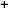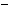Labware - MA35 Multivariable Calculus - Polar Coordinate Labs
 MA35 Labs » Polar Coordinate Labs Contents.1 Functions of Two Variables .1.1 Introduction .1.2 Domain, Range & Function Graphs .1.3 Slice Curves .1.5 Continuity Search

Domain, Range & Function Graphs

Text

The domain of a function of two variables in polar coordinates is a subset of the polar coordinate plane { (r,θ) | r ∈ R \mbox{and} 0 {leq} θ < 2π.The range of a real-valued function f is the collection of all real numbers f(p) where p is in the domain of f.The graph of a function of two variables is the collection of points (x,y,f(x,y)) in 3-space where (x,y) is in the domain of f. When we write the domain in polar coordinates, the graph is said to be in cylindrical coordinates.

Demos

 Domain and RangeThis demonstration graphs a function f[r,θ] over a disc domain. By default, the domain is set to 0 ≤ r ≤ 1, -π ≤ θ ≤ π and the function is set to f[r,θ] = r2cos(2θ). In the window labeled Domain and Range, you can choose both the center and radius of a red disc domain in the xy-plane using the white and red hotspots respectively. The magenta line segment along the z-axis in this window shows the range of f[r.θ] over the red disc domain.Exercises

• What is the range of the function f(x,y) = -x4 + 2x2 - y2 over a unit disc domain centered at the origin (i.e. the set of all points (x,y) such that 0 ≤ r ≤ 1)?
• Describe the graph of f(x,y) = 2xy/(x2 + y2) for all values of x, y other than (0,0) where the function is not defined.